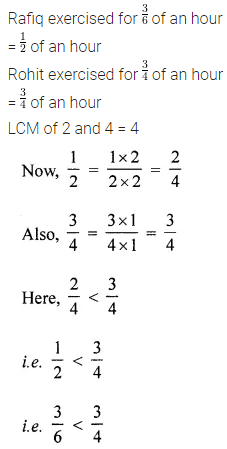# ML Aggarwal Class 6 Solutions for ICSE Maths Chapter 6 Fractions Ex 6.7

## ML Aggarwal Class 6 Solutions for ICSE Maths Chapter 6 Fractions Ex 6.7

Question 1.
Sarita bought $$\frac{2}{5}$$ metre of ribbon and Laiita $$\frac{3}{4}$$ metre of ribbon. What is the total length of the ribbon they bought?
Solution: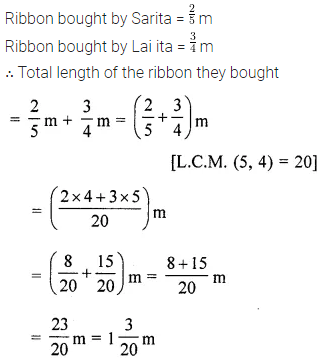Question 2.
A bamboo of length $$2 \frac{3}{4}$$ metre broke into two pieces. One-piece was $$\frac{7}{8}$$ metre long. How long is the other piece?
Solution: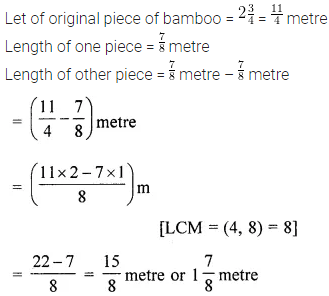Question 3.
Nidhi’s house is $$1 \frac{9}{10}$$ km from her school. She walked some distance and then took a bus for $$1 \frac{1}{2}$$ km to reach the school. How far did she walk?
Solution: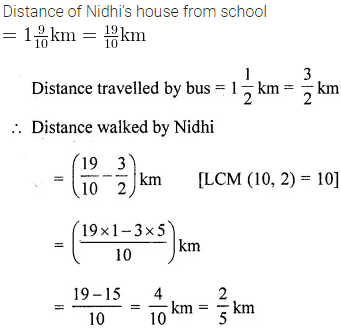Question 4.
From a rope of length $$20 \frac{1}{2}$$ m, a piece of length $$3 \frac{5}{8}$$ m is cut off. Find the length of the remaining rope.
Solution: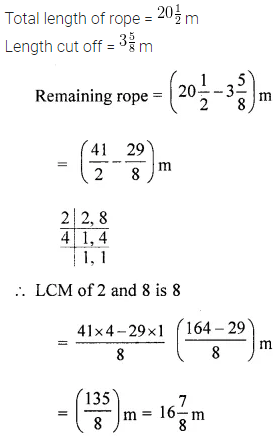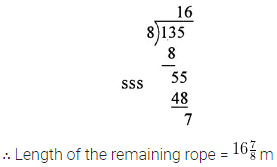Question 5.
The weight of three packets are $$2 \frac{3}{4}$$ kg. $$3 \frac{1}{3}$$ kg. and $$5 \frac{2}{5}$$ kg. Find total weight of all the three packets.
Solution:Question 6.
Shivani read 25 pages of a book containing 100 pages. Nandni read $$\frac{2}{5}$$ of the same book. Who reads less?
Solution:Question 7.
Rafiq exercised for $$\frac{3}{6}$$ of an hour, while Rohit, exercised for $$\frac{3}{4}$$ of an hour. Who exercised for a longer time and by what fraction of an hour?
Solution: